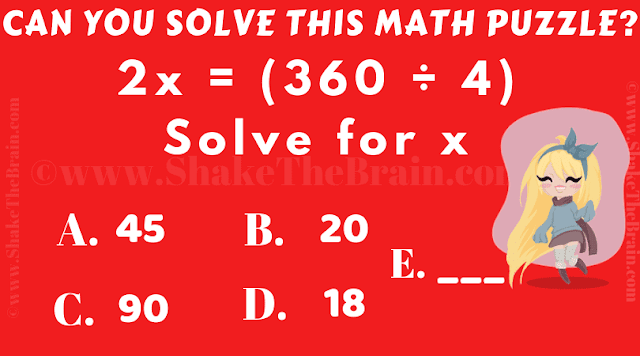Someone wanted to test your quick calculations in Maths. Do remember to solve this Maths puzzle as quickly as possible as one has to solve this equation while driving. So can you find the value of X?Can you solve this math puzzle?

Answer of this "Quick Maths Picture Puzzle", can be viewed by clicking on the answer button.